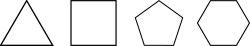# Perimeter facts for kids

Kids Encyclopedia FactsPerimeter is the distance around a two dimensional shape, a measurement of the distance around something; the length of the boundary.

In geometry, perimeter is the distance around a flat object. For example, all four sides of a square rhombus have the same length, so a rhombus with side length 2 inches would have a perimeter of 8 inches (2+2+2+2=8).

Real-life objects have perimeters as well. A football field, including the end zones, is 360 feet long and 160 feet wide. So the perimeter of the field is 360+160+360+160=1040 feet.

The perimeter of a circle is usually called the circumference. It may be calculated by multiplying the diameter times "Pi". Pi is a constant which is equal to 3.14159; however, the places to the right of the decimal are endless. The number of places used depend on the accuracy required for the result.

## Images for kidsPerimeter Facts for Kids. Kiddle Encyclopedia.